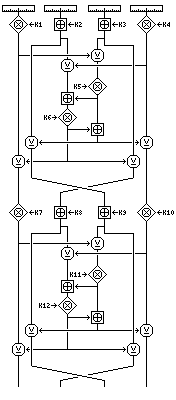[Next] [Up] [Previous] [Index]

IDEA (International Data Encryption Algorithm)

IDEA, unlike the other block cipher algorithms discussed in this section, is patented by the Swiss firm of Ascom. They have, however, been generous in allowing, with permission, free noncommercial use of their algorithm, with the result that IDEA is best known as the block cipher algorithm used within the popular encryption program PGP.

The IDEA algorithm is interesting in its own right. It includes some steps which, at first, make it appear that it might be a non-invertible hash function instead of a block cipher. Also, it is interesting in that it entirely avoids the use of any lookup tables or S-boxes.

IDEA uses 52 subkeys, each 16 bits long. Two are used during each round proper, and four are used before every round and after the last round. It has eight rounds.

The plaintext block in IDEA is divided into four quarters, each 16 bits long. Three operations are used in IDEA to combine two 16 bit values to produce a 16 bit result, addition, XOR, and multiplication. Addition is normal addition with carries, modulo 65,536. Multiplication, as used in IDEA, requires some explanation.

Multiplication by zero always produces zero, and is not invertible. Multiplication modulo n is also not invertible whenever it is by a number which is not relatively prime to n. The way multiplication is used in IDEA, it is necessary that it be always invertible. This is true of multiplication IDEA style.

The number 65,537, which is 2^16+1, is a prime number. (Incidentally, 2^8+1, or 257, is also prime, and so is 2^4+1, or 17, but 2^32+1 is not prime, so IDEA cannot be trivially scaled up to a 128-bit block size.) Thus, if one forms a multiplication table for the numbers from 1 through 65,536, each row and column will contain every number once only, forming a Latin square, and providing an invertible operation. The numbers that 16 bits normally represent are from 0 to 65,535 (or, perhaps even more commonly, from -32,768 to 32,767). In IDEA, for purposes of multiplication, a 16 bit word containing all zeroes is considered to represent the number 65,536; other numbers are represented in conventional unsigned notation, and multiplication is modulo the prime number 65,537.

Description of IDEA

Let the four quarters of the plaintext be called A, B, C, and D, and the 52 subkeys called K(1) through K(52).

Before round 1, or as the first part of it, the following is done:

Multiply A by K(1). Add K(2) to B. Add K(3) to C. Multiply D by K(4).

Round 1 proper consists of the following:

Calculate A xor C (call it E) and B xor D (call it F).

Multiply E by K(5). Add the new value of E to F.

Multiply the new value of F by K(6). Add the result, which is also the new value of F, to E.

Change both A and C by XORing the current value of F with each of them; change both B and D by XORing the current value of E with each of them.

Swap B and C.

Repeat all of this eight times, or seven more times, using K(7) through K(12) the second time, up to K(43) through K(48) the eighth time. Note that the swap of B and C is not performed after round 8.

Then multiply A by K(49). Add K(50) to B. Add K(51) to C. Multiply D by K(52).

The intricacies of IDEA encryption may be made somewhat clearer by examining the following diagrams:

Details:Overview:Decryption

How can the round in IDEA be reversed, since all four quarters of the block are changed at the same time, based on a function of all four of their old values? Well, the trick to that is that A xor C isn't changed when both A and C are XORed by the same value, that value cancels out, no matter what that value might be. And the same applies to B xor D. And since the values used are functions of (A xor C) and (B xor D), they are still available.

This cross-footed round, rather than a Feistel round, is the most striking distinguishing factor of IDEA, although its use of multiplication, addition, and XOR to avoid the use of S-boxes is also important.

Those that are added are replaced by their two's complement. Those that are multiplied in are replaced by their multiplicative inverse, modulo 65,537, in IDEA notation when used to change blocks directly, but those used to calculate the cross-footed F-functions are not changed. Keys XORed in would not need to be changed, but there aren't any such keys in IDEA. Due to the placement of the swap, the first four keys for decryption are moved somewhat differently than the other keys used for the same operation between rounds.

The decryption key schedule is:

The first four subkeys for decryption are:

KD(1) = 1/K(49)
KD(2) =  -K(50)
KD(3) =  -K(51)
KD(4) = 1/K(52)
and they do not quite follow the same pattern as the remaining subkeys which follow.

The following is repeated eight times, adding 6 to every decryption key's index and subtracting 6 from every encryption key's index:

KD(5)  =   K(47)
KD(6)  =   K(48)

KD(7)  = 1/K(43)
KD(8)  =  -K(45)
KD(9)  =  -K(44)
KD(10) = 1/K(46)

Subkey generation

The 128-bit key of IDEA is taken as the first eight subkeys, K(1) through K(8). The next eight subkeys are obtained the same way, after a 25-bit circular left shift, and this is repeated until all encryption subkeys are derived.

This method of subkey generation is regular, and this may be a weakness. However, IDEA is considered to be highly secure, having stood up to all forms of attack so far tried by the academic community.

[Next] [Up] [Previous] [Index]